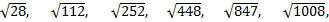# Important Quantitative Aptitude Questions for IBPS CLERK & RBI ASSISTANT MAINS – SET 3

Dear bankersdaily Aspirant,

Quantitative Aptitude section was the second most attempted section after the reasoning section in the recently over IBPS CLERK Prelims and RBI Assistant Prelims Exam 2017. Also many of the aspirants have been facing difficulties in attempting the questions from this particular section as the questions asked in this section varies for exam to exam and not constant.

So we have decide to provide daily questions based on each topic available in the Quantitative Aptitude section and the aspirants can get benefited and get accustomed to the pattern of the questions in the quants section. The timing required to solve  those questions should be less which the aspirants will gain in solving all these questions.

Try these questions which are asked in the recent examinations and check your level of expertise in these topics and this could also be useful for your forthcoming exams too.

Find the missing number in the following number series

Q.1) 35, 60, 110, 260, 860, ?

(a) 4230

(b) 3480

(c) 3860

(d) 3280

(e) 3560

Find the missing number in the following number series

Q.2) 2,  10,  29,  70,  144,  ?

(a) 198

(b) 251

(c) 262

(d) 217

(e) 273

Find the missing number in the following number series

Q.3) 11, 22,  66,  264,  1320,  ?

(a) 7160

(b) 7920

(c) 7840

(d) 7660

(e) 7940

Find the missing number in the following number series

Q.4) ?, 10, 10, 20, 80, 640

(a) 5

(b) 30

(c) 10

(d) 40

(e) 20

Find the missing number in the following number series

Q.5) 12,  16,  25,  50, ?, 258

(a) 144

(b) 125

(c) 114

(d) 80

(e) 156

In each of these questions a number series is given. In each series only one number is wrong. Find out the wrong number.

Q.6)(a) √28

(b) √112

(c) √252

(d) √448

(e) √847

In each of these questions a number series is given. In each series only one number is wrong. Find out the wrong number.

Q.7) 19,     748,   1619, 2681, 3983, 5574, 7508

(a) 748

(b) 2681

(c) 5574

(d) 19

(e) 7508

In each of these questions a number series is given. In each series only one number is wrong. Find out the wrong number.

Q.8) 711,   728,   863,   1206, 1935, 3266

(a) 3266

(b) 1935

(c) 728

(d) 711

(e) 863

In each of these questions a number series is given. In each series only one number is wrong. Find out the wrong number.

Q.9) 6,       10,     39,     103,   437,   2221, 13375

(a) 10

(b) 39

(c) 103

(d) 6

(e) 2221

In each of these questions a number series is given. In each series only one number is wrong. Find out the wrong number.

Q.10) 628,   863,   1478, 2703, 4728, 7753, 11978

(a) 628

(b) 1478

(c) 4728

(d) 863

(e) 7753

Check the Answers for the above questions from the link given below

Explanation

Q.1)

c

35          60            110             260            860            3860

25           50            150              600            3000

Q.2)

c

2           10           29            70            144              262

8            19            41             74              118

11           22             33              44

Q.3)

b

11           22            66            264            1320            7920

×2          ×3            ×4              ×5              ×6

Q.4)

e

20             10           10              20            80           640

×0.5           ×1            ×2             ×4           ×8

Q.5)

c

12            16             25              50             114             258

22              32              52                82              122

1               2                3                 4

Q.6)

e

The series is,

2√7,  4√7,  6√7,  8√7,  10√7, 12√7,

Hence, √847 is the wrong number.

Q.7)

e

The difference is,

729,            871,            1062,          1302,          1591,          1934

142,            191,            240,            289,            343

49,              49,              49,              54

Hence, 7508 is the wrong number.

Q.8)

c

The difference is,

27,     125,   343,   729,   1331

Hence, 728 is the wrong number.

Q.9)

b

The series is,

×1+22, ×2+32, ×3+42, ×4+52, ×5+62, ×6+72,

Hence, 39 is the wrong number.

Q.10)

d

The difference is,

225,   625,   1225, 2025, 3025, 4225

Hence, 863 is the wrong number.

You can check the other Important Reasoning Topics below here.